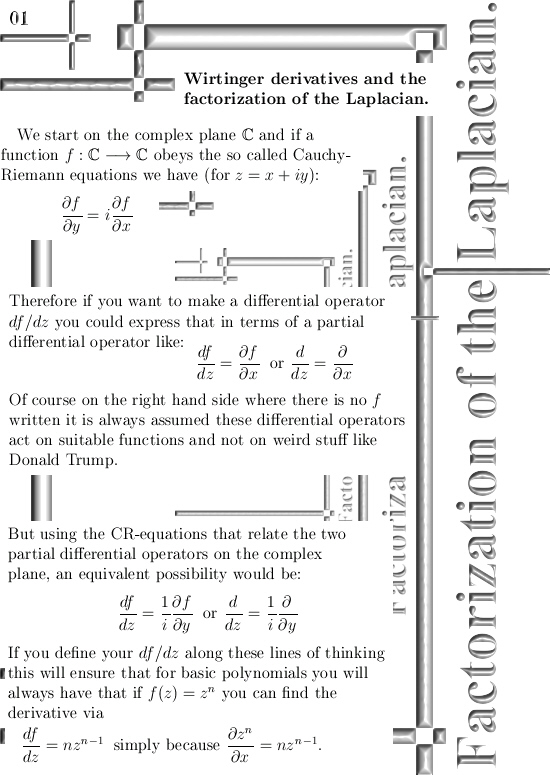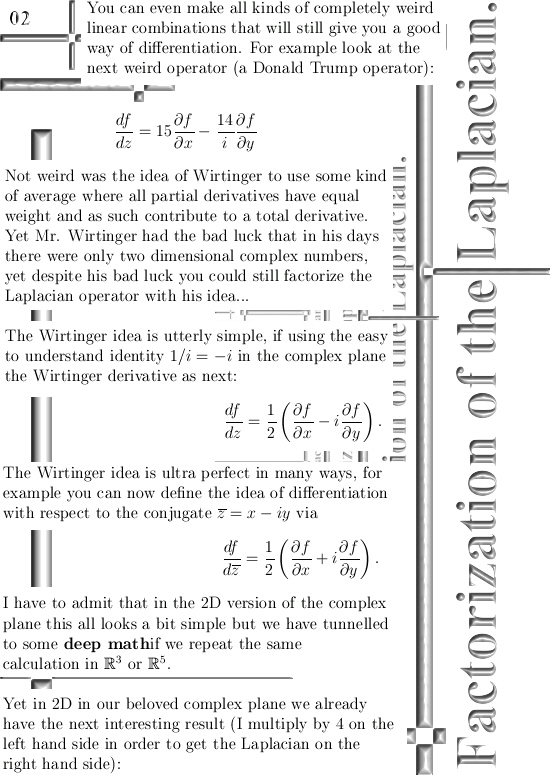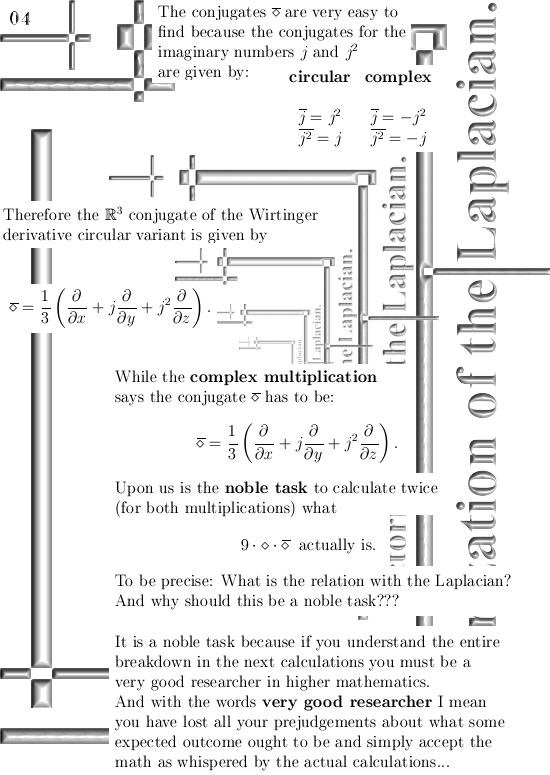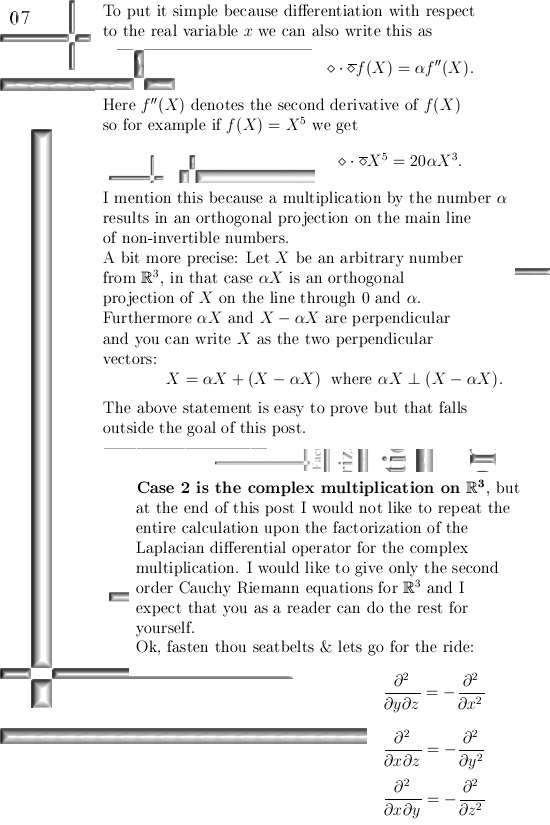# Wirtinger derivatives and the factorization of the Laplacian.

This post could have many titles, for example ‘Factorization of the Laplacian using second order Cauchy-Riemann equations’ would also cover what we will read in the next seven pictures.

The calculation as shown below is, as far as I am concerned, definitely in the top ten of results relating to all things 3D complex numbers. Only when I stumbled on this a few years back I finally understood the importance of the so called sphere-cone equation.

The calculation below is basically what you do when writing out the sphere-cone equation only now it is not with variables like x, y and z but with the partial differential operators with respect to x, y and z. In simplifying the expressions we get I use so called second order Cauchy-Riemann equations, if you understand the standard CR equations these second order equations are relatively easy to digest.This post is also categorized under Quantum Mechanics, the reason for that is that the wave equation contains the Laplacian operator and the more you know about that rather abstract thing the better it is in my view.

I would like to close with a link to a wiki on Wirtinger derivatives, originally they come from theory with several complex variables. That explains why in the wiki the Wirtinger derivatives are written as partial derivatives while above we can use the straight d´s for our differential of f.

Here is the wiki: Wirtinger derivatives
https://en.wikipedia.org/wiki/Wirtinger_derivatives